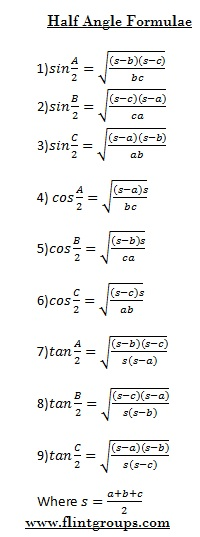# TRIGONOMETRY : Properties of Triangles

## Properties of Triangles

Consider a triangle ABC.it has three angles A,B and C.The sides opposite to the angles A,B,C are denoted by the corresponding small letters a,b,c respectively.
Thus a=BC,b=CA,c=AB in the fig. shown below.Sine Formulaa/sina  = b/sinB=c/sinc=2R  Where R,is a Radius of Circle circum circle of the triangle.

Napier's Formulae :Cosine Formulae:

1. a2=b2+c2 -2bc cosA
2. b2=c2+a2 -2ca cosB
3. c2=a2+b2 -2ab cosC
Projection Formulae :
1. a =bcosC+ccosB
2. b=ccosA+acosC
3. c=acosB+bcosA
Half Angle Formulae :Area Formulae: